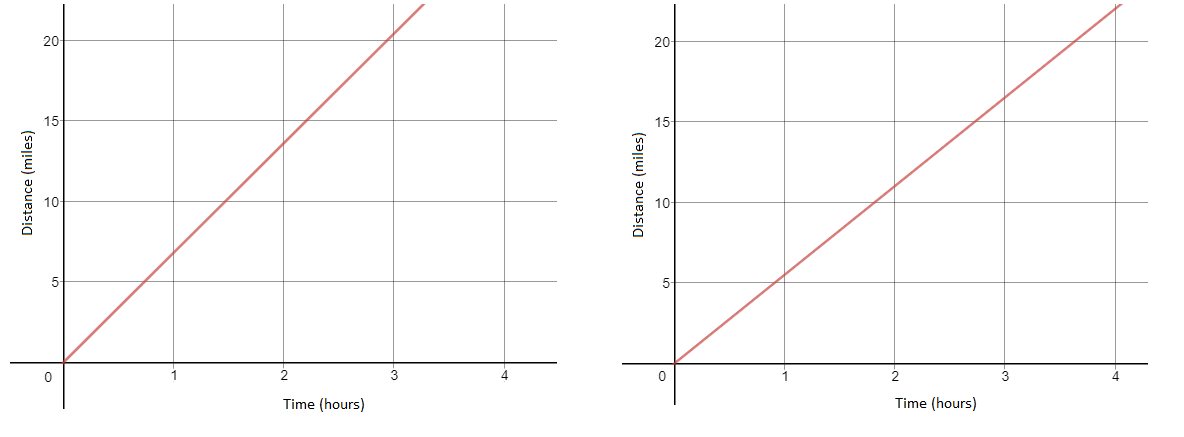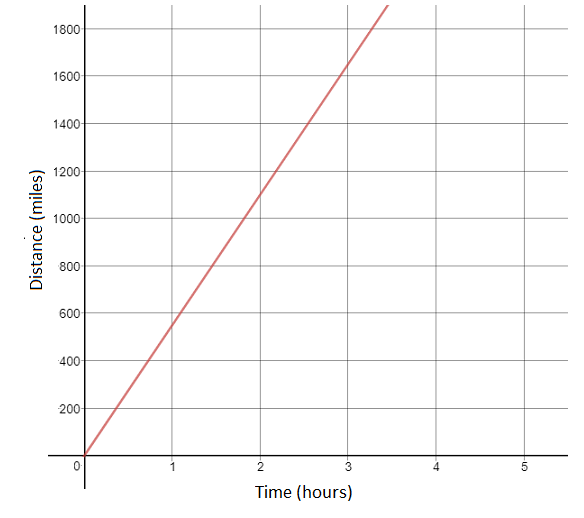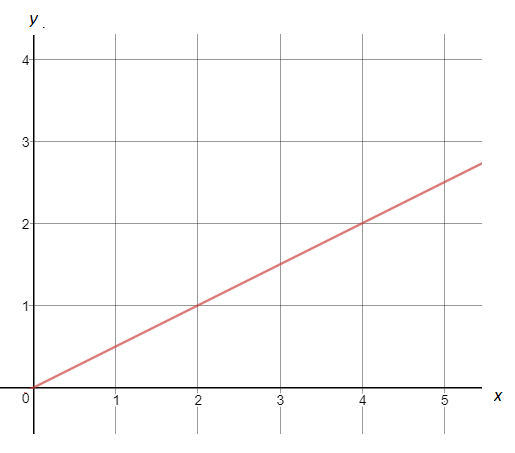# Linear Relationships

## Objective

Review representations of proportional relationships.

## Common Core Standards

### Core Standards

?

• 8.EE.B.5 — Graph proportional relationships, interpreting the unit rate as the slope of the graph. Compare two different proportional relationships represented in different ways. For example, compare a distance-time graph to a distance-time equation to determine which of two moving objects has greater speed.

?

• 7.RP.A.2

## Criteria for Success

?

1. Represent proportional situations involving speed as equations, graphs, tables, and descriptions.
2. Understand that the constant of proportionality is the same as the rate of change in a proportional relationship.
3. Understand that the rate of change in a situation is the same as the slope of the graph that represents the situation.

## Tips for Teachers

?

• This lesson is approaching 8.EE.5 and reviews concepts from seventh grade Ratios and Proportions standards and from Unit 4 of eighth grade using the context of speed. In Lesson 2, students will draw graphs of proportional relationships and continue to make connections between the different representations. This will prepare students for Lessons 3 and 4, where students will compare across proportional relationships.
• The term slope is introduced in this lesson. Students learn that the rate of change is the same as the slope of the graph. The concept of slope will be developed more thoroughly in later lessons in the unit.

#### Remote Learning Guidance

This lesson reviews concepts from 7th grade; Anchor Problems can be chosen based on what specific concept and/or skill review students need. Find more guidance on adapting our math curriculum for remote learning here.

#### Fishtank Plus

• Problem Set
• Student Handout Editor
• Vocabulary Package

## Anchor Problems

?

### Problem 1

Asia is training for a 10K road race that will take place in October. She starts her training in July and runs every weekend. On her first run, she ran at a constant pace and covered 5.5 miles in 60 minutes. On her last run before the race, she ran at a constant pace and covered 10.2 miles in 1 ½ hours.

1. What were Asia’s speeds, in miles per hour, on her first and last runs?
2. Write an equation to represent Asia’s distance ran as a function of time for her first run and for her last run.
3. A 10K road race is approximately 6.2 miles. If Asia ran at her beginning pace, how long would it take her to finish the road race? How long would it take her if she ran at her end pace?
4. Determine which graph below represents Asia’s first run and her last run.### Problem 2

The graph below shows the relationship between the distance an airplane has covered and the amount of time it has been flying.1. What is the speed of the airplane in miles per hour?
2. What is the rate of change in the relationship?
3. What is the slope of the graph?
4. What equation represents the relationship?

## Problem Set

?The following resources include problems and activities aligned to the objective of the lesson that can be used to create your own problem set.

• Include problems where students are given a proportional graph and asked to find the slope of the graph (or rate of change in situation) and write the equation.

?

Four representations are shown below. Three of the four representations match.

Which one does not belong? Explain your reasoning.

Graph:Equation:

${y=2x}$

Table:

 $x$ 2 4 6 8 $y$ 1 2 3 4

Description:

A caterpillar moves at a constant speed of $\frac{1}{2}$ inch per second. Let $x$ represent the time in seconds and $y$ represent the distance traveled in inches.

?